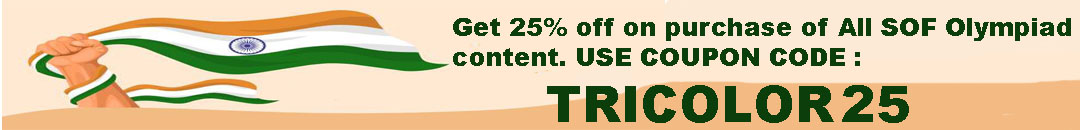# User ForumSubject :IMO    Class : Class 4

Class : Class 1

The correct answer is option C.

## Ans 2:

Class : Class 5
for me i did c but it said wrong

Class : Class 5
CC

## Ans 4:

Class : Class 5
C is the the correct answer.

## Ans 5:

Class : Class 2
maybe you thought you pressed C but you pressed some other one by mistake.

Subject :IMO    Class : Class 4

Class : Class 1

The correct answer is option B.

## Ans 2:

Class : Class 4
The correct answer is B option

## Ans 3:

Class : Class 7

Subject :IMO    Class : Class 4

Class : Class 1

(C):

Total number of votes polled = 83457
Votes polled in favour of one candidate = 49682
Let the number of votes polled in favour of other candidate be X.
Number of invalid votes = 2974
So, 83457 = 49682 + X + 2974
83457 = 52656 + X

X = 83457 – 52656
X = 30801
Therefore, the number of votes polled in favour of other candidate is 30801.

Subject :IMO    Class : Class 2

Class : Class 2

## Ans 2:

Class : Class 3
dont you have any sense in your brain i am going to take your brain and eat it

Class : Class 4

## Ans 4:

Class : Class 3
Answer is option (C), 6. Thanks. :D

## Ans 5:

Class : Class 3
Explaination : Answer is option (c) because 1st Step - The small 4 squares. 2nd Step - The large full square. 3rd Step - The square in which the small squares are included.

## Ans 6:

Class : Class 4
The correct answer is option C.

## Ans 7:

Class : Class 3
is the tilted square inside the main outer square not counted as square. With this one, there are six squares in total.

Class : Class 4
c

Class : Class 2

## Ans 10:

Class : Class 4

Subject :IMO    Class : Class 6

Class : Class 1

(D): Number of cubes in top layer = 1
Number of cubes in middle layer = 5
Number of cubes in bottom layer = 9
Total number of cubes = 1 + 5 + 9 = 15

Subject :IMO    Class : Class 2

## Ans 1:

Class : Class 3

Subject :IMO    Class : Class 1

Subject :IMO    Class : Class 1

Subject :IMO    Class : Class 3

Class : Class 4
D